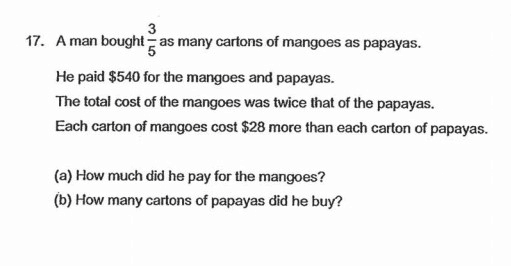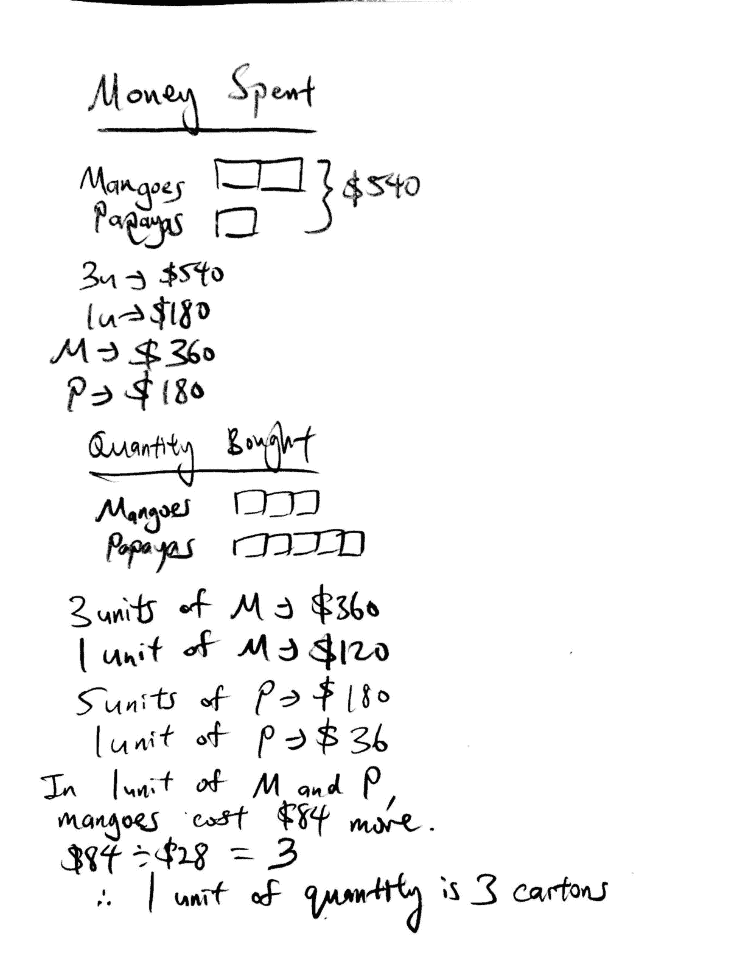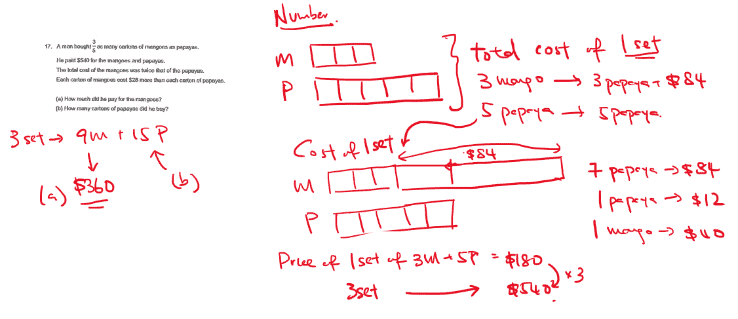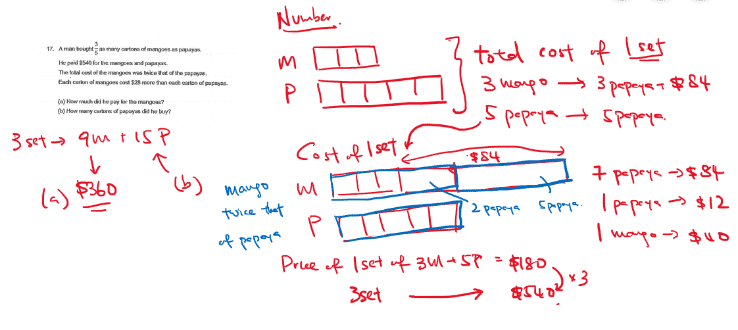# QuestionHi can anyone help with this? Thank you!Hi KateM let me try to help you with this question as well.

First of all, yes that is right, this question is similar to the one that you have posted. Let me try to explain the concept and method under the contexts of this question. Hopefully it will be helpful!

We know that the amount of money spent on the fruits is as such, as seen in my workings:

\$360 on mangoes, \$180 on papayas.

So to answer part a) of the question, it is \$360. This is fairly simple. Part b) might be a little more challenging. (:

As we know, the number of cartons bought for mangoes will be 3 units, while papaya will be 5 units. If we were to compare 1 unit of each type of fruit, it would mean that the quantity of fruits will be the same. (1 unit of mango and 1 unit of papaya)

So, we know that 3 units of mangoes (quantity) will cost \$360, 1 unit of mangoes (quantity) will cost \$120.

We know that 5 units of papayas (quantity) will cost \$180, 1 unit of papayas (quantity) will cost \$36.

Now, the same number of mangoes and papayas are being compared (1 unit each), and we see that the mangoes cost \$120 – \$36 = \$84 more.

Knowing that one carton of mango costs \$28 more than a carton of papaya, this means that if we take 84 divided by 28, we will get 3. And, 3 represents the number of cartons per unit of fruit (quantity)

You see, let’s backtrack a little, let’s look at 1 unit of mango and 1 unit of papaya. If say, there were 1 carton of mango and 1 carton of papaya, it would mean that the mangoes costs \$28 dollars more.

If say, there were 2 cartons of mangoes and 2 cartons of papayas, it would mean that the mangoes costs \$56 more.

If say, there were 3 cartons of mangoes and 3 cartons of papayas, it would mean that the mangoes costs \$84 dollars more (the precise number we are looking at!)

So, see, this is why when we take 84 divided by 28, we get 3.

Now knowing that 1 unit of fruit represents 3 cartons, to answer part b), it would be 3 multiplied by 5 units of papayas, that would give me 15.

Hope this helps! Feel free to PM me (:

http://www.futureachievers.com.sg

9114 3397

Thank you for the clear explanation!

0 Replies 0 Likesgot to guide your child with comparison model and use the set/group method to solve this question. definitely not the easiest question so first spend time to guide your child with the two model drawings to establish the cost of 1 papaya and 1 mango. After which use the set/group method to find out how many papayas and mangoes are there. Guide your child with these two thinking processes!

Hope this helps! We covered this type of question extensively in our website

www.ensoeducate.com/sgcourses

Thank you ensoeducation for the answer

May i ask how do you get 7 papayas equals to \$84? I am a little confused

Also, one mango is twice the cost of one papaya. One papaya is \$12, shouldnt the mango be \$24 in this case?